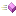The following tables list the members exposed by EllipticCurveCalculator.

 Name DescriptionThis is Add, a member of class EllipticCurveCalculator.This is EllipticCurveCalculator, a member of class EllipticCurveCalculator.This is InverseMod, a member of class EllipticCurveCalculator.This is Modular, a member of class EllipticCurveCalculator.This is PointNeg, a member of class EllipticCurveCalculator.This is ScalarMult, a member of class EllipticCurveCalculator.